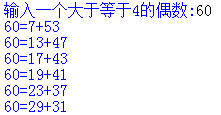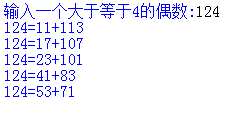# python哥德巴赫猜想,python利用该函数验证哥德巴赫猜想-Python

实例1输入一个大于等于4的偶数60

60=7+53

60=13+47

60=17+43

60=19+41

60=23+37

60=29+31